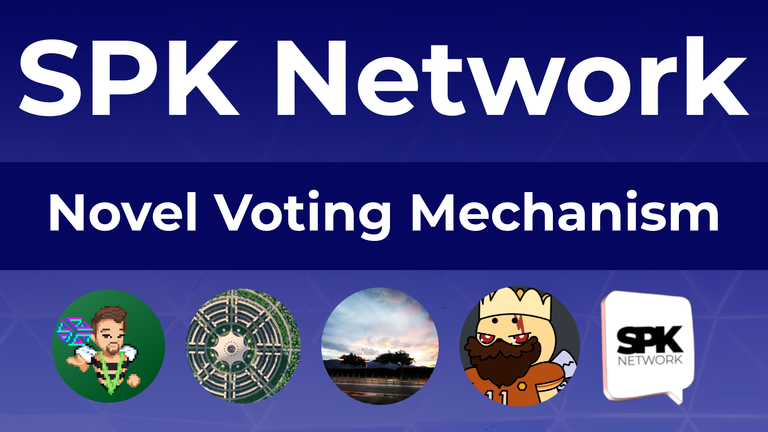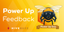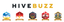# Novel Voting Mechanism - SPK Network Team Meeting

in SPK Network2 months agoWe're buttoning up the next iteration of the SPK Network. Before we get there, we're discussing our new voting mechanism and hoping to get your feedback. This meeting is approaching an hour long, and below is all the code referenced directly in the video... with some additional //comments.

``````exports.spk_vote = (json, from, active, pc) => { //the contract json:payload, from:@hiveaccount, active: active key used, pc:promise chain(for contract ordering)
var ops = [] //holds raw memory instructions
if (active) { //ensures active key
var powp = getPathNum(["spow", from]), // @from's powered spk
tpowp = getPathNum(["spow", "t"]), // the total powered spk
dpowp = getPathObj(["spowd", from]), // @from's downpower operations pointer
votebp = getPathObj(['spkVote', from]), // @from's last vote information
pstats = getPathNum(['stats']) // current network parameters
Promise.all([powp, tpowp, dpowp, votebp, pstats]).then((mem) => {
var stats = mem
const DAOString = mem.substring(mem.indexOf(",")),
lastVote = Base64.toNumber(mem.split(","))
? Base64.toNumber(mem.split(","))
: json.block_num - parseInt(stats.spk_cycle_length),
thisVote =
Base64.fromNumber(json.block_num) + "," + (DAOString ? DAOString : ""), //decoding terse memory
ago = json.block_num - lastVote
total = mem,
power = mem
downs = Object.keys(mem)
var effective_power = power, effective_total, aValidator = false
if(stats.validators?.[from]){ //determine if @from is a validator
aValidator = true
var powerVoted = 0
for (block of stats.power_voted){
powerVoted += stats.power_voted[block]
}
power = (total - powerVoted)/20 //or number of validators
}
if (!power){
ops.push({
type: "put",
path: ["feed", `\${json.block_num}:\${json.transaction_id}`],
data: `@\${from}| Attempted SPK vote with no voting power`,
});
store.batch(ops, pc);
} else if(downs.length && !aValidator){
getPathObj(['chrono', downs]).then(down =>{ // additional step to recover downpower information from pointer
finish(down)
})
} else {
finish()
}
function finish(down_obj) {
if(down_obj?.amount){
effective_power = power - down_obj.amount
}
if (ago < parseFloat(stats.spk_cycle_length))effective_power = parseInt(effective_power * (ago / stats.spk_cycle_length))
else if (ago > parseFloat(stats.spk_cycle_length) && ago < stats.spk_cycle_length * 2)effective_power = effective_power* parseInt(
effective_power *
(1 - ((ago - stats.spk_cycle_length) / stats.spk_cycle_length) / 2)
)
else if (ago >= stats.spk_cycle_length * 2)effective_power = parseInt(effective_power/2)
effective_total = effective_total - effective_power
const voteWeight = parseFloat(effective_power/effective_total).toFixed(8)
const decayWeight = parseFloat(
(effective_total - effective_power) / effective_total
).toFixed(8);
if(json.spk_cycle_length < 28800)json.spk_cycle_length = 28800
if(json.spk_cycle_length > 3000000)json.spk_cycle_length = 3000000
if(json.dex_fee < 0)json.dex_fee = 0
if(json.dex_fee > 0.1)json.dex_fee = "0.1"
if(json.dex_max < 0)json.dex_max = 0
if(json.dex_max > 100)json.dex_max = 100
if(json.dex_slope < 0)json.dex_slope = 0
if(json.dex_slope > 100)json.dex_slope = 100
if(json.spk_rate_lpow < 0)json.spk_rate_lpow = 0
if(json.spk_rate_lpow > stats.spk_rate_ldel)json.spk_rate_lpow = stats.spk_rate_ldel
if(json.spk_rate_ldel > stats.spk_rate_lgov)json.spk_rate_lpow = stats.spk_rate_lgov
if(json.spk_rate_ldel < stats.spk_rate_lpow)json.spk_rate_ldel = stats.spk_rate_lpow
if(json.spk_rate_lgov > 0.1)json.spk_rate_lgov = "0.1"
if(json.spk_rate_lgov < stats.spk_rate_ldel)json.spk_rate_lpow = stats.spk_rate_ldel
if(json.max_coll_members > 100)json.max_coll_members = 100
if(json.max_coll_members < 15)json.max_coll_members = 15
json.max_coll_members = parseInt(json.max_coll_members)
//stats.item = (json.vote * voteWeight) + (decayWeight * stats.item)
stats.spk_cycle_length = (json.spk_cycle_length * voteWeight) + (decayWeight * parseFloat(stats.spk_cycle_length)) > 28800 ? parseFloat((json.spk_cycle_length * voteWeight) + (decayWeight * stats.spk_cycle_length).toFixed(6)) : 28800
stats.dex_fee = parseFloat((json.dex_fee * voteWeight) + (decayWeight * parseFloat(stats.dex_fee))).toFixed(6)
stats.dex_max = parseFloat((json.dex_max * voteWeight) + (decayWeight * parseFloat(stats.dex_max))).toFixed(2)
stats.dex_slope = parseFloat((json.dex_slope * voteWeight) + (decayWeight * parseFloat(stats.dex_slope))).toFixed(2)
stats.spk_rate_ldel = parseFloat((json.spk_rate_ldel * voteWeight) + (decayWeight * parseFloat(stats.spk_rate_ldel))).toFixed(6)
stats.spk_rate_lgov = parseFloat((json.spk_rate_lgov * voteWeight) + (decayWeight * parseFloat(stats.spk_rate_lgov))).toFixed(6)
stats.spk_rate_lpow = parseFloat((json.spk_rate_lpow * voteWeight) + (decayWeight * parseFloat(stats.spk_rate_lpow))).toFixed(6)
stats.max_coll_members = (json.max_coll_members * voteWeight) + (decayWeight * parseFloat(stats.max_coll_members)) < 25 ? 25 : ((json.max_coll_members * voteWeight) + (decayWeight * stats.max_coll_members) > 79 ? 79 : parseFloat((json.max_coll_members * voteWeight) + (decayWeight * stats.max_coll_members)).toFixed(6))
if(!aValidator)stats.power_voted[stats.lastIBlock] = effective_power + (typeof stats.power_voted[stats.lastIBlock] == "number" ? stats.power_voted[stats.lastIBlock] : 0)
ops.push({
type: "put",
path: ["stats"],
data: stats,
});
ops.push({
type: "put",
path: ["spkVote", from],
data: thisVote,
});
ops.push({
type: "put",
path: ["feed", `\${json.block_num}:\${json.transaction_id}`],
data: `@\${from}| Has updated their votes.`,
});
store.batch(ops, pc);
}
});
} else {
ops.push({
type: "put",
path: ["feed", `\${json.block_num}:\${json.transaction_id}`],
data: `@\${from}| Attempted SPK vote with posting key`,
});
store.batch(ops, pc);
}
}

``````

Thank you for participating in our development process and participating in these early stages.

#### Vote for our Witness:The SPK Network is a decentralized Web 3.0 protocol that rewards value creators and infrastructure providers appropriately and autonomously by distributing reward tokens so that every user, creator, and platform, will be able to earn rewards on a level playing field.

▶️ 3Speak

2 months ago (edited)

The rewards earned on this comment will go directly to the people( @yeckingo1 ) sharing the post on Twitter as long as they are registered with @poshtoken. Sign up at https://hiveposh.com.

Congratulations @spknetwork! You have completed the following achievement on the Hive blockchain And have been rewarded with New badge(s)You published more than 40 posts.Your next target is to reach 50 posts.

If you no longer want to receive notifications, reply to this comment with the word `STOP`

Check out our last posts:Our Hive Power Delegations to the January PUM WinnersFeedback from the February Hive Power Up DayThe Hive Gamification Proposal
###### Support the HiveBuzz project. Vote for our proposal!

is really hard to follow, sound is not that clear :)

2 months ago  Reveal Comment

You're mostly right here, but at the end of the day I don't think it matters. Staking didn't stop the Sun takeover, and median values are exceptionally unlikely to be ideal values. The moving average here is done to give the most number of people the ability to vote, as a system that calculates median values would need to store and calculate all accounts votes at every vote, which won't scale. What seems like a median on Hive really is the median of 20 accounts who have a few key votes, a barely decentralized plutocracy.

The number of people who have left Hive is just shy of all them. Let's not stretch our imagination too much and think 90% of accounts hold 20% of the stake. On Hive there is nothing they could do even together to get a single witness voted from outside the top. At least with this system they could exercise 20% of the vote toward governance. The top 20 in this paradigm are the people who vote for all the apathetic accounts... which would have prevented the Sun takeover. It also disallows the biggest accounts from exercising more than 5%(hopefully closer to 2.5% with 50% apathy) votes as well, which addresses the last point: Any single vote won't effect a variable more than 5% in the arbitrary range.

The code does have vote range limits, so a negative vote will just be counted as a minimum. At this stage at least most of the range limits are fairly natural. Interest rates can't be negative, key holders are limited by Hive code, power-down voting range is something like 1 to 100 days... (which translates to 4 to 400 days for a full powerdown). The flip side is the 13 week power down on Hive is almost kinda voted on when there is a hardfork... but as much as people want it to drop in line with the rest of the market, there isn't even a path to do so.

To sum

Pros:

• Scalable
• Non-exclusionary
• Same or better whale influence limit as Hive.
• Same of better arbitrary vote range limits as Hive

Cons:

• Less plutocratic(?)